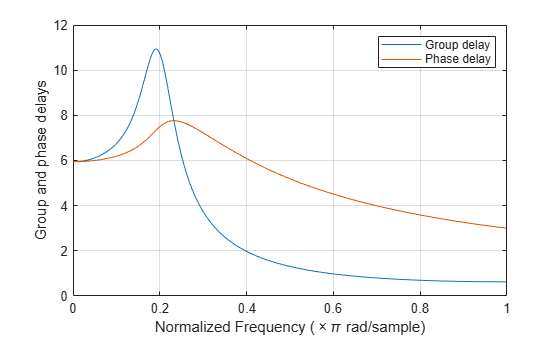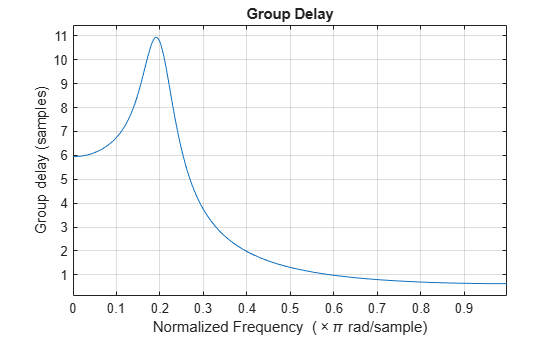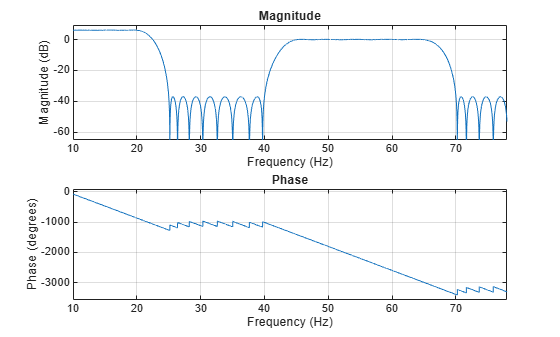# grpdelay

Average filter delay (group delay)

## Syntax

[gd,w] = grpdelay(b,a,n)
[gd,w] = grpdelay(sos,n)
[gd,w] = grpdelay(d,n)
[gd,w] = grpdelay(___,'whole')
[gd,f] = grpdelay(___,n,fs)
[gd,f] = grpdelay(___,n,'whole',fs)
gd = grpdelay(___,win)
gd = grpdelay(___,fin,fs)
grpdelay(___)

## Description

[gd,w] = grpdelay(b,a,n) returns the n-point group delay response vector, gd, and the corresponding angular frequency vector, w, for the digital filter with transfer function coefficients stored in b and a.

example

[gd,w] = grpdelay(sos,n) returns the n-point group delay response corresponding to the second-order sections matrix sos.

example

[gd,w] = grpdelay(d,n) returns the n-point group delay response for the digital filter d.
[gd,w] = grpdelay(___,'whole') returns the group delay at n sample points around the entire unit circle.
[gd,f] = grpdelay(___,n,fs) returns the group delay response vector gd and the corresponding physical frequency vector f for a digital filter designed to filter signals sampled at a rate fs.
[gd,f] = grpdelay(___,n,'whole',fs) returns the frequency vector at n points ranging between 0 and fs.
gd = grpdelay(___,win) returns the group delay response vector gd evaluated at the normalized frequencies supplied in win.

example

gd = grpdelay(___,fin,fs) returns the group delay response vector gd evaluated at the physical frequencies supplied in fin.

example

grpdelay(___) with no output arguments plots the group delay response of the filter.

## Examples

collapse all

Design a Butterworth filter of order 6 with normalized 3-dB frequency $0.2\pi$ rad/sample. Use grpdelay to display the group delay.

[z,p,k] = butter(6,0.2); sos = zp2sos(z,p,k); grpdelay(sos,128)Plot both the group delay and the phase delay of the system on the same figure.

gd = grpdelay(sos,512); [h,w] = freqz(sos,512); pd = -unwrap(angle(h))./w; plot(w/pi,gd,w/pi,pd) grid xlabel 'Normalized Frequency (\times\pi rad/sample)' ylabel 'Group and phase delays' legend('Group delay','Phase delay')Use designfilt to design a sixth-order Butterworth Filter with normalized 3-dB frequency $0.2\pi$ rad/sample. Display its group delay response.

d = designfilt('lowpassiir','FilterOrder',6, ... 'HalfPowerFrequency',0.2,'DesignMethod','butter'); grpdelay(d)Design an 88th-order FIR filter of arbitrary magnitude response. The filter has two passbands and two stopbands. The lower-frequency passband has twice the gain of the higher-frequency passband. Specify a sample rate of 200 Hz. Visualize the magnitude response and the phase response of the filter from 10 Hz to 78 Hz.

fs = 200; d = designfilt('arbmagfir', ... 'FilterOrder',88, ... 'NumBands',4, ... 'BandFrequencies1',[0 20], ... 'BandFrequencies2',[25 40], ... 'BandFrequencies3',[45 65], ... 'BandFrequencies4',[70 100], ... 'BandAmplitudes1',[2 2], ... 'BandAmplitudes2',[0 0], ... 'BandAmplitudes3',[1 1], ... 'BandAmplitudes4',[0 0], ... 'SampleRate',fs); freqz(d,10:1/fs:78,fs)Compute and display the group delay response of the filter over the same frequency range. Verify that it is one-half of the filter order.

filtord(d)
ans = 88 
grpdelay(d,10:1/fs:78,fs)## Input Arguments

collapse all

Transfer function coefficients, specified as vectors. Express the transfer function in terms of b and a as

$H\left({e}^{j\omega }\right)=\frac{B\left({e}^{j\omega }\right)}{A\left({e}^{j\omega }\right)}=\frac{\text{b(1)}+\text{b(2)}\text{\hspace{0.17em}}{e}^{-j\omega }+\text{b(3)}\text{\hspace{0.17em}}{e}^{-j2\omega }+\cdots +\text{b(M)}\text{\hspace{0.17em}}{e}^{-j\left(M-1\right)\omega }}{\text{a(1)}+\text{a(2)}\text{\hspace{0.17em}}{e}^{-j\omega }+\text{a(3)}\text{\hspace{0.17em}}{e}^{-j2\omega }+\cdots +\text{a(N)}\text{\hspace{0.17em}}{e}^{-j\left(N-1\right)\omega }}.$

Example: b = [1 3 3 1]/6 and a = [3 0 1 0]/3 specify a third-order Butterworth filter with normalized 3 dB frequency 0.5π rad/sample.

Data Types: double | single
Complex Number Support: Yes

Number of evaluation points, specified as a positive integer scalar no less than 2. When n is absent, it defaults to 512. For best results, set n to a value greater than the filter order.

Second-order section coefficients, specified as a matrix. sos is a K-by-6 matrix, where the number of sections, K, must be greater than or equal to 2. If the number of sections is less than 2, the function treats the input as a numerator vector. Each row of sos corresponds to the coefficients of a second-order (biquad) filter. The ith row of sos corresponds to [bi(1) bi(2) bi(3) ai(1) ai(2) ai(3)].

Example: s = [2 4 2 6 0 2;3 3 0 6 0 0] specifies a third-order Butterworth filter with normalized 3 dB frequency 0.5π rad/sample.

Data Types: double | single
Complex Number Support: Yes

Digital filter, specified as a digitalFilter object. Use designfilt to generate a digital filter based on frequency-response specifications.

Example: d = designfilt('lowpassiir','FilterOrder',3,'HalfPowerFrequency',0.5) specifies a third-order Butterworth filter with normalized 3 dB frequency 0.5π rad/sample.

Sample rate, specified as a positive scalar. When the unit of time is seconds, fs is expressed in hertz.

Data Types: double

Angular frequencies, specified as a vector and expressed in rad/sample. win must have at least two elements, because otherwise the function interprets it as n. win = π corresponds to the Nyquist frequency.

Frequencies, specified as a vector. fin must have at least two elements, because otherwise the function interprets it as n. When the unit of time is seconds, fin is expressed in hertz.

Data Types: double

## Output Arguments

collapse all

Group delay response, returned as a vector. If you specify n, then gd has length n. If you do not specify n, or specify n as the empty vector, then gd has length 512.

If the input to grpdelay is single precision, the function computes the group delay using single-precision arithmetic. The output h is single precision.

Angular frequencies, returned as a vector. w has values ranging from 0 to π. If you specify 'whole' in your input, the values in w range from 0 to 2π. If you specify n, w has length n. If you do not specify n, or specify n as the empty vector, then w has length 512.

Frequencies, returned as a vector expressed in hertz. f has values ranging from 0 to fs/2 Hz. If you specify 'whole' in your input, the values in f range from 0 to fs Hz. If you specify n, f has length n. If you do not specify n, or specify n as the empty vector, then f has length 512.

collapse all

### Group Delay

The group delay response of a filter is a measure of the average delay of the filter as a function of frequency. It is the negative first derivative of the phase response of the filter. If the frequency response of a filter is H(e), then the group delay is

${\tau }_{g}\left(\omega \right)=-\frac{d\theta \left(\omega \right)}{d\omega },$

where θ(ω) is the phase, or argument, of H(e).

## Version History

Introduced before R2006a